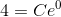# AP Calculus BC : Modeling by Solving Separable Differential Equations

## Example Questions

### Example Question #1 : Modeling By Solving Separable Differential Equations

Solve the separable differential equation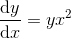with the condition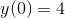.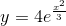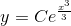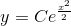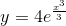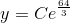Explanation:

To solve the separable differential equation, we must separate x and y, dx and dy respectively to opposite sides: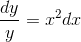Integrating both sides, we get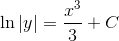The rules of integration used were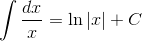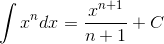The constants of integration merged into one.

Now, we exponentiate both sides of the equation to solve for y, and use the properties of exponents to simplify: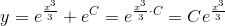To solve for C, we use our given condition: